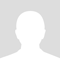# The ordering of algebraic operations

When both writing down and reading the algebraic expressions, the binary operation (including addition+, subtraction-, multiply*, divide/, exponential^) follow a conventional order:

0) Parenthesis, including {}, [], ()
1) Exponent, multiply and divide

The ordering is 0)>1)>2). Then there is no ordering within each group, eg multiply and divide are at the same level of priority except that 0) comes in such as a parenthesis.

Let's take a look at one quick example: 3+(8-2)*6.
First compute (8-2)=6;
Then compute (8-2)*6=6*6=36;
Finally compute 3+(8-2)*6=3+36=39.

Another example: 3^2+3/(5-2)
First compute (5-2)=3;
Then do 3/(5-3)=3/3=1;
Next compute 3^2=3*3=9;

Hope it helps!